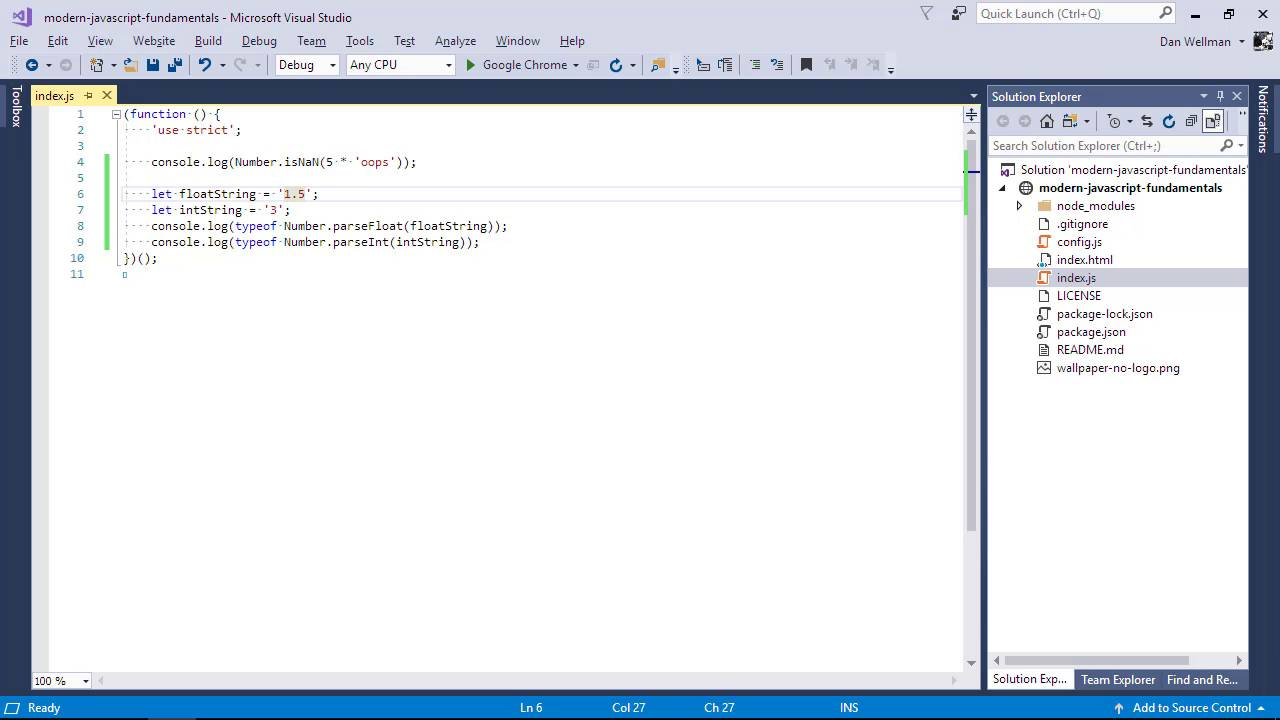Lessons: 65Length: 7.1 hours• Overview
• Transcript

# 5.3 Working With Numbers

In this lesson we’ll take a look at some of the properties and methods that make up the API of the built-in Number object.

Syntax

• isNaN
• parseFloat
• parseInt
• toFixed
• toExponential
• toPrecision
• toString

## 11.Conclusion1 lesson, 00:43

### 5.3 Working With Numbers

Hey folks, in this lesson we're going to look at numbers. Like strings, numbers are another type of primitive. And also like strings, they have a number of properties and methods for working with them. And that's what we're going to focus on today. The number object contain some properties that present different values in JavaScript. Like max_value, min_value, max_safe_integer, and min_safe_integer, which are the maximum and minimum values and safe values that it is possible to use in JavaScript. There are also some very useful methods. There is a special number in JavaScript called NaN, which is produced when we try to do something with a number which is not possible. Like multiplying it by a string. The problem with NaN values is that they ruin any mathematical calculations that you are trying to perform. And they are very hard to detect. Because NaN is not equal to anything, not even itself, so this makes it very hard to test for in a conditional. To overcome this, we have the isNaN method, which we can use to detect when a value is NaN. Let's take a look. And lets check the output now in our browser. And we can see that, that has resulted in the value of true. So, isNaN is a static method which is invoked directly on the number object rather than on number instances. We also have these static methods isFinite, isInteger, and isSafeInteger for performing others checks on numbers. The last two static methods are very commonly used. If we want to convert strings into numbers, there are two more static methods that we can make use of. parseFloat for parsing floating point numbers from strings, and parseInt, for parsingintegers. Let's take a look at how these two work. So here we've defined two strings which happen to contain numbers. Let's convert them directly into numbers instead of strings. So they look like numbers, but let's use the typeof operator just to be certain. And we can see that they are both numbers. The strings that we're parsing from don't have to just contain numbers, they can also contain numbers and letters. And these two methods should still work in the same way depending on where the numbers occur. And we can see that we have still managed to extract the numbers from those strings, even though the strings contain more than just numbers. However, in order to parse numbers successfully from strings, the numbers need to come at the start of the string. So when we add non numerical numbers to the start of a string, before any numbers occur, and try to parse those numbers out of it, we'll get the value not a number. And just to highlight how dangerous the not a number or NAN value is, let's just go back and add typeof again to the start of the first console log. And it misleadingly tells us that we're still working with a number, because the NAN value is a type of number. So we have to be very careful when parsing numbers from strings to make sure that we don't end up with this NAN value. As well as the static methods that we've looked at so far, number instances also have several methods that can be used to work with the number in some way. For example, to convert a number to have a specific number of decimal places, we can use the toFixed method So we've invoked the toFixed method on our number instance, and we've passed it the argument 2. So it should truncate the number down to two decimal places. Let's just check the output in our browser. And as you can see, that has worked. And the rounding that has occurred is probably what you would expect from regular mathematics. As well as toFixed, we also have toExponential, toLocaleString, toPrecision, and toString methods. The toFixed and toPrecision methods are very similar. The difference between them is that toFixed rounds to a fixed number of decimal places while toPrecision formats a number to a given number of significant digits. So in this lesson we looked at numbers. We saw the static properties and methods that we can use from the number object directly. And we also saw some of the instance methods that we can use with number instances. In the next lesson, we'll move on to look at how we can work with arrays. Thanks for watching.

Back to the top